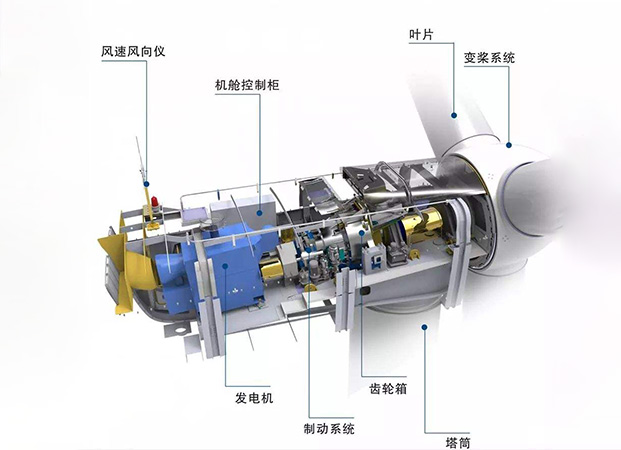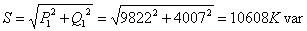# 补偿电容器在大型风电主风机组补偿无功案例

2020-07-20 09:03 库克库伯57P1=2300/0.947=2429kW

Q1=P1xtg∮=2429x0.763=1853kvar

cos∮n=0.90时，tg∮=0.4843

Q1=9822x0.4843=4757kvar

Q1=Q1-Q=4757-750=4007kvar,cos∮=9822/10608=0.926